High-Performance Solenoid Drives

by Pete Millett, Sr. Technical Marketing Engineer, Monolithic Power Systems

Solenoids are used in many applications to provide linear or rotational actuation in mechanical systems. Although driving solenoids can be as simple as switching the current flow on and off, oftentimes better performance can be obtained using a dedicated IC to drive them.

In this article, we will examine how drive circuitry affects the electromechanical performance of solenoids. Two different drive circuits will be compared: a simple switch and a current regulating driver. Energy-saving techniques that limit power dissipation in the solenoid will also be illustrated.

Solenoid Basics

In its simplest form, a solenoid is a coil of wire that generates a magnetic field. The devices we usually refer to as solenoids are devices that use a coil of wire and a moving core made of iron or sometimes another magnetic material. Applying current to the coil causes the core to be pulled or pushed relative to the coil, causing motion that is used to actuate something in a mechanical system. A typical solenoid is shown in Figure 1.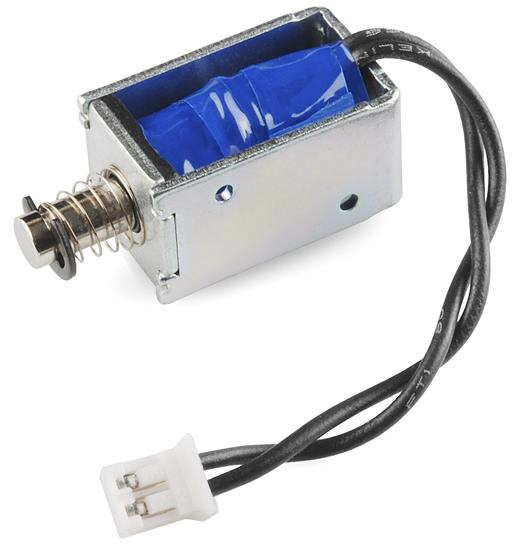Figure 1: A Typical Solenoid

When actuating a solenoid, a voltage is applied to the winding to create a magnetic field. Since the winding has a large inductance, the current takes some time to build up. The force on the core of the solenoid is proportional to the current. To generate maximum force to move the core, a high voltage must be applied to the winding to build the current quickly.

Once the motion is complete, a much smaller current usually can be used to hold the core in position. If the current is not reduced, considerable power is dissipated in the winding, and the solenoid generates a great amount of heat.

To solve these problems, a constant-current driver can be used to drive the solenoid. The current can be controlled over time to provide ideal actuation and limit the power dissipated to hold the solenoid in place.

Test Set-Up

To compare the electromechanical performance of different solenoid drive schemes, a simple test set-up was built using a servo potentiometer connected to a solenoid with a flexure to allow the motion of the solenoid to be measured. Motion, along with voltage and current, were captured using an oscilloscope. An MPS MPQ6610 IC was used to drive the solenoid. The set-up is shown in Figure 2.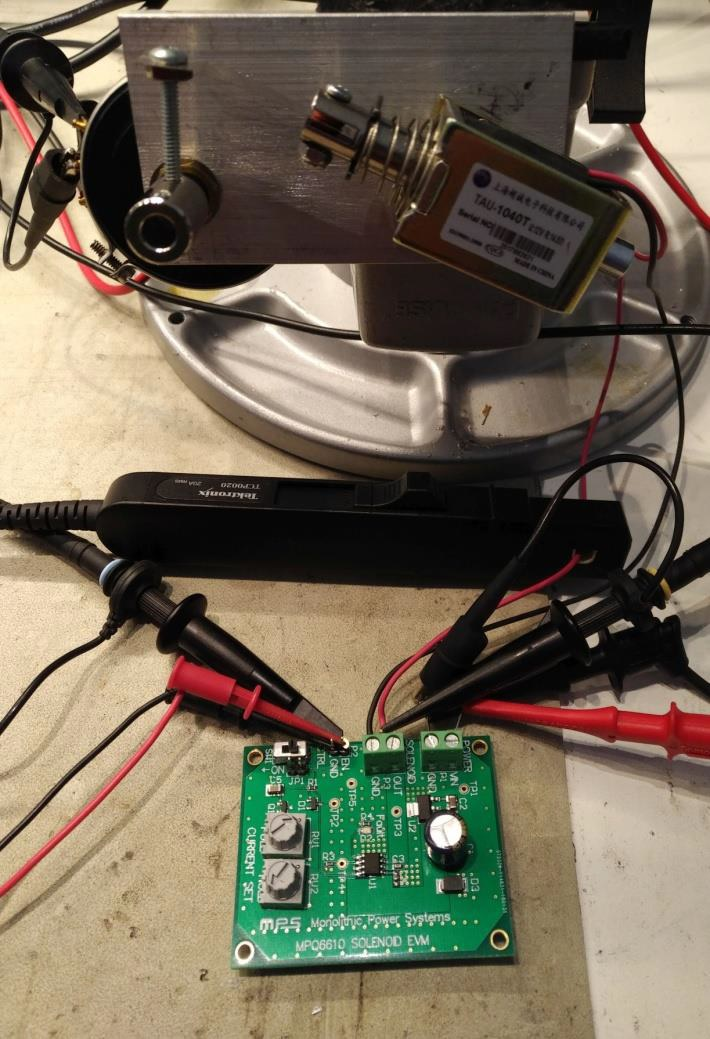Figure 2: Test Set-Up

Simple Solenoid Drivers

The simplest way to drive a solenoid is to simply switch the current on and off. This is often done with a low-side MOSFET switch and current recirculation diode (see Figure 3). In this circuit, the current is limited only by the supply voltage and DC resistance of the solenoid.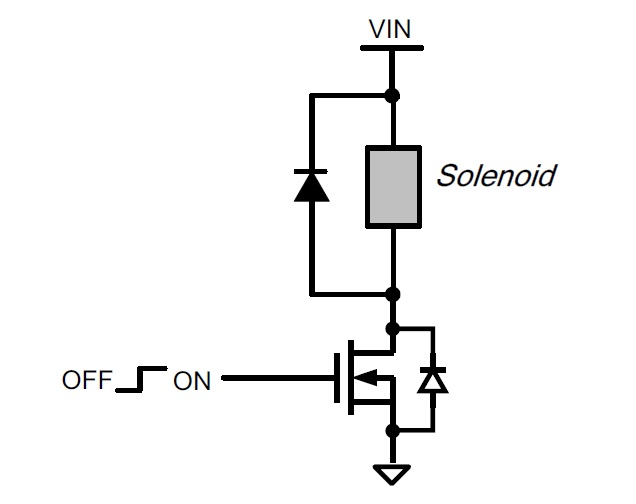Figure 3: Simple Solenoid Driver

The electromechanical performance of a simple drive is limited. Since the full voltage and current are applied 100% of the time, the pull-in current is constrained by the continuous power dissipation rating of the solenoid. The large inductance of the coil also limits how quickly the current can increase when the solenoid is first activated.

>In our test, the motion, voltage, and current of the solenoid using a simple switch was measured (see Figure 4). In this case, the solenoid (15Ω, rated for 12V) took 30ms to actuate and dissipated 10W of power whenever the solenoid was actuated.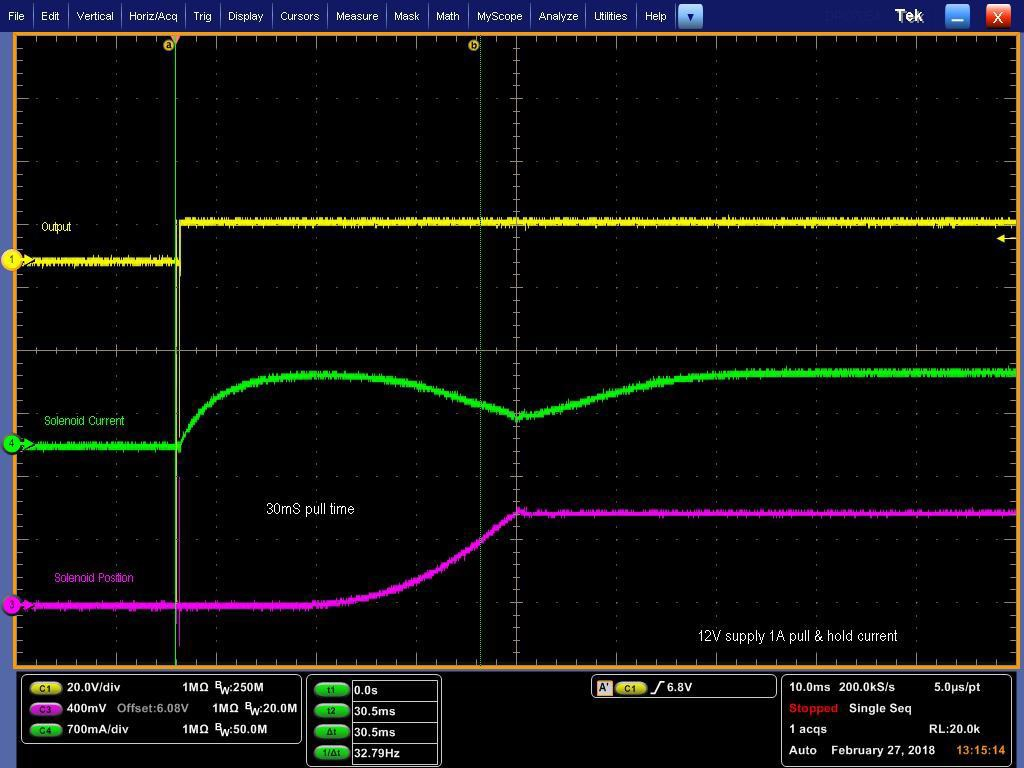Figure 4: Simple Switch Waveforms

>If you are wondering about the “valley” in the current waveform, this reduction in current is due to the back EMF generated by the moving core of the solenoid. The back EMF increases as the core accelerates, until the point that the solenoid bottoms out and stops moving.

A High-Performance Solenoid Driver

In most applications, full current is only needed initially to pull in the solenoid. After the motion is complete, the current level in the solenoid can be lowered, which results in energy savings and much less heat generated in the coil. This also allows a higher supply voltage to be used, which provides a higher pull-in current to make the solenoid actuate faster and provide more force.

An MPS MPQ6610 half-bridge driver with a few external components can accomplish this (see Figure 5). The MPQ6610 is a rated for up to 60V and 3A and is available in small TSOT and SOIC packages.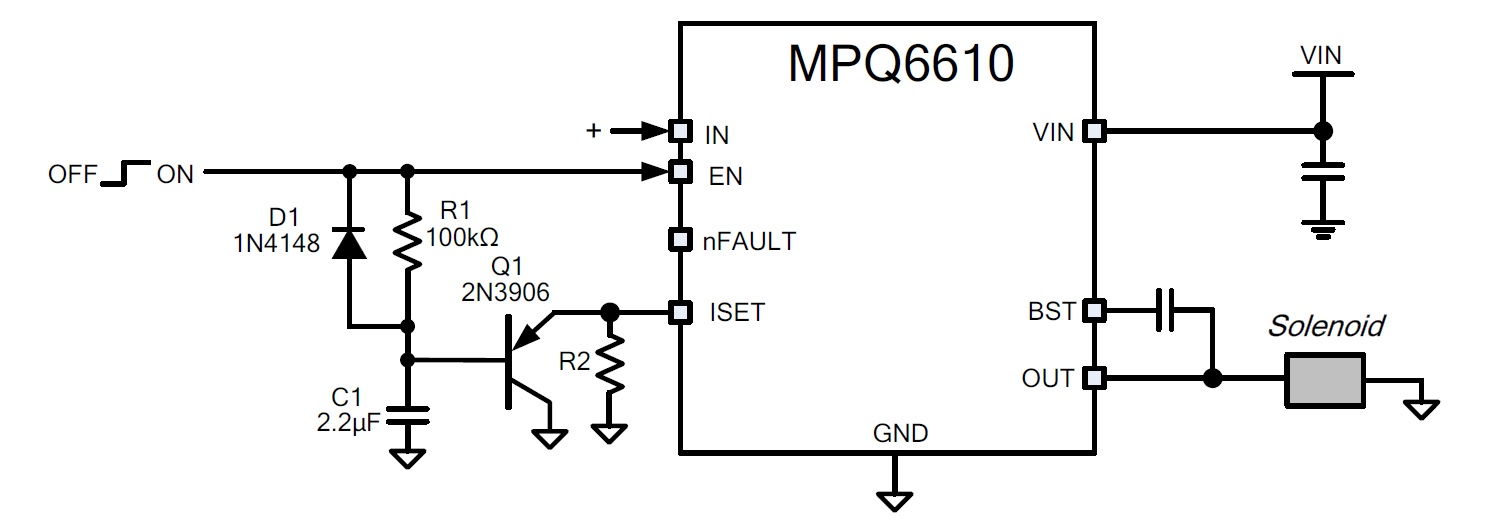Figure 5: MPQ6610 Reduced Hold Current Circuit

The resulting drive waveforms are shown in Figure 6. The yellow trace is the OUT signal driving the solenoid, and the green trace is the solenoid current. Initially, the full supply voltage (24V in this case) is driven to pull in the solenoid. After a delay, the current is reduced by pulse-width modulating the output. The pull-in time is reduced to 16ms, and the holding power dissipation is much lower (around 600mW instead of 10W).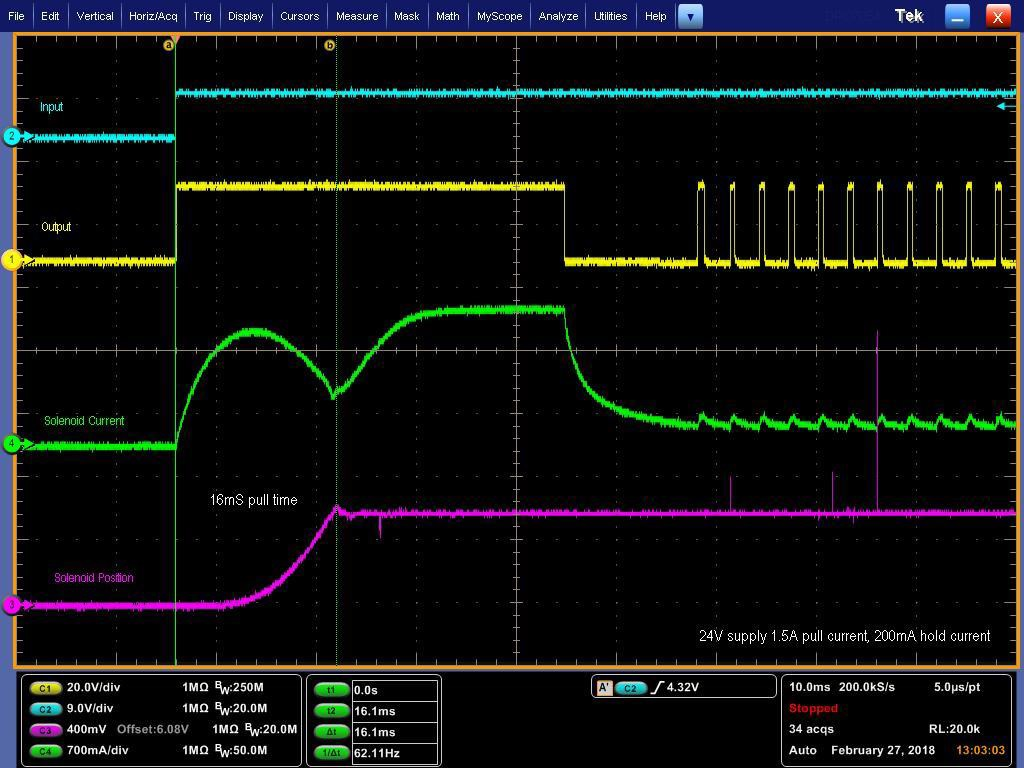Figure 6: Reduced Hold Current Waveforms

This circuit works like this: Initially, the input signal is low. This discharges C1 through D1, and holds the ISET pin low through Q1. The input signal goes high, which enables the MPQ6610 and drives the output high, applying the full supply voltage to the solenoid. C1 starts charging through R1. Current is sourced from the ISET pin proportional to the current flowing in the solenoid. As C1 charges, the voltage on the ISET pin is allowed to rise.

Assuming there is sufficient current flowing in the solenoid, the voltage on the ISET pin continues rising until it reaches its current regulation threshold (1.5V). At this point, the MPQ6610 starts regulating the solenoid current. The regulated hold current is set by the value of R2.

The delay time (where the solenoid is driven at 100% duty cycle) is set by the values of R1 and C1. For a standard 3.3V logic level, the time is approximately 0.33 x RC. For the example above with R1 = 100kΩ and C1 = 2.2μF, 0.33 x RC = 75mS.

For additional information, refer to the MPQ6610 datasheet and application notes at www.monolithicpower.com.

Conclusion

The measurements presented here show that improved performance and much lower energy consumption can be attained using a current-regulating driver to drive solenoids. Small IC drivers, such as the MPS MPQ6610, provide this performance benefit at a small cost and require very little PCB area.

_________________________